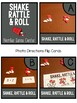# Number Sense Centers for Composing and Decomposing Numbers with LolaSubject
Resource Type
File Type

Zip

(30 MB|365 pages)
Product Rating
Standards
• Product Description
• StandardsNEW

Students will

•Construct an understanding of numbers through hands-on work.

•Discover constancy & patterns while working with one number, then the next.

•Describe the parts of many different number arrangements.

•Determine the whole when the parts of a number are known.

•Determine a missing part of a number when the other part is known.

•Find all the ways a number can be decomposed into two or more parts.

Students can work at the following levels, ensuring that each student is working at the level right where they need to be. These different levels can also be used for different grade levels.

Concept Level

•FIRST build models with concrete materials

•THEN describe what they built with mathematical language

Connecting Level

•EITHER build concrete models to match written equations

•OR match equations to their model

Symbolic Level

•FIRST build concrete models

•THEN record/write equations to match what they’ve built

Add and subtract within 20, demonstrating fluency for addition and subtraction within 10. Use strategies such as counting on; making ten (e.g., 8 + 6 = 8 + 2 + 4 = 10 + 4 = 14); decomposing a number leading to a ten (e.g., 13 - 4 = 13 - 3 - 1 = 10 - 1 = 9); using the relationship between addition and subtraction (e.g., knowing that 8 + 4 = 12, one knows 12 - 8 = 4); and creating equivalent but easier or known sums (e.g., adding 6 + 7 by creating the known equivalent 6 + 6 + 1 = 12 + 1 = 13).
Apply properties of operations as strategies to add and subtract. If 8 + 3 = 11 is known, then 3 + 8 = 11 is also known. (Commutative property of addition.) To add 2 + 6 + 4, the second two numbers can be added to make a ten, so 2 + 6 + 4 = 2 + 10 = 12. (Associative property of addition.)
Decompose numbers less than or equal to 10 into pairs in more than one way, e.g., by using objects or drawings, and record each decomposition by a drawing or equation (e.g., 5 = 2 + 3 and 5 = 4 + 1).
Represent addition and subtraction with objects, fingers, mental images, drawings, sounds (e.g., claps), acting out situations, verbal explanations, expressions, or equations.
Total Pages
365 pages
N/A
Teaching Duration
1 Year
Report this Resource to TpT
Reported resources will be reviewed by our team. Report this resource to let us know if this resource violates TpT’s content guidelines.